# Introduction to quantum mechanics

Introduction to quantum mechanics

Quantum mechanics is the body of scientific principles which attempts to explain the behavior of matter and its interactions with energy on the scale of atoms and atomic particles.

Just before 1900, it became clear that classical physics was unable to model certain phenomena. Coming to terms with these limitations led to the development of quantum mechanics, a major revolution in physics. This article describes how the limitations of classical physics were discovered, and the main concepts of the quantum theories which replaced them in the early decades of the 20th century.[note 1] These concepts are described in roughly the order they were first discovered; for a more complete history of the subject, see History of quantum mechanics.

Some aspects of quantum mechanics can seem counter-intuitive, because they describe behavior quite different than that seen at larger length scales, where classical physics is an excellent approximation. In the words of Richard Feynman, quantum mechanics deals with "nature as she is — absurd."

Many types of energy, such as photons (discrete units of light), behave in some respects like particles and in other respects like waves. Radiators of photons (such as neon lights) have emission spectra which are discontinuous, in that only certain frequencies of light are present. Quantum mechanics predicts the energies, the colours, and the spectral intensities of all forms of electromagnetic radiation.

But quantum mechanics theory ordains that the more closely one pins down one measure (such as the position of a particle), the less precise another measurement pertaining to the same particle (such as its momentum) must become. Put another way, measuring position first and then measuring momentum does not have the same outcome as measuring momentum first and then measuring position; the act of measuring the first property necessarily introduces additional energy into the micro-system being studied, thereby perturbing that system.

Even more disconcerting, pairs of particles can be created as entangled twins — which means that a measurement which pins down one property of one of the particles will instantaneously pin down the same or another property of its entangled twin, regardless of the distance separating them — though this may be regarded as merely a mathematical anomaly, rather than a real one.

## The first quantum theory: Max Planck and black body radiationHot metalwork from a blacksmith. The yellow-orange glow is the visible part of the thermal radiation emitted due to the high temperature. Everything else in the picture is glowing with thermal radiation as well, but less brightly and at longer wavelengths than the human eye can detect. A far-infrared camera will show this radiation.

Thermal radiation is electromagnetic radiation emitted from the surface of an object due to the object's temperature. If an object is heated sufficiently, it starts to emit light at the red end of the spectrum — it is red hot. Heating it further causes the colour to change, as light at shorter wavelengths (higher frequencies) begins to be emitted. It turns out that a perfect emitter is also a perfect absorber. When it is cold, such an object looks perfectly black, because it absorbs all the light that falls on it and emits none. Consequently, an ideal thermal emitter is known as a black body, and the radiation it emits is called black body radiation.

In the late 19th century, thermal radiation had been fairly well-characterized experimentally. The wavelength at which the radiation is strongest is given by Wien's displacement law, and the overall power emitted per unit area is given by the Stefan–Boltzmann law. Therefore, as temperature increases, the glow colour changes from red to yellow to blue to white. Even as the peak wavelength moves into the ultra-violet, enough radiation continues to be emitted in the blue wavelengths that the body continues to appear blue. It never becomes invisible—indeed, the radiation of visible light increases monotonically with temperature. Physicists were searching for a theoretical explanation for these experimental results.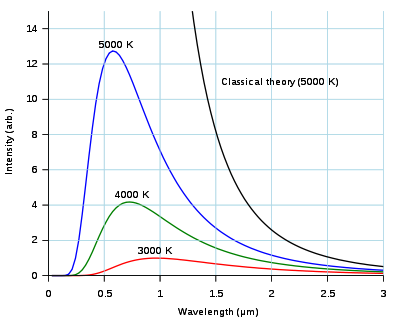The peak wavelength and total power radiated by a black body vary with temperature. Classical electromagnetism drastically overestimates these intensities, particularly at short wavelengths.

The "answer" found using classical physics is the Rayleigh–Jeans law. This law agrees with experimental results at long wavelengths. At short wavelengths, however, classical physics predicts that energy will be emitted by a hot body at an infinite rate. This result, which is clearly wrong, is known as the ultraviolet catastrophe.

The first model which was able to explain the full spectrum of thermal radiation was put forward by Max Planck in 1900. He modeled the thermal radiation as being in equilibrium, using a set of harmonic oscillators. To reproduce the experimental results he had to assume that each oscillator produced an integral number of units of energy at its one characteristic frequency, rather than being able to emit any arbitrary amount of energy. In other words, the energy of each oscillator was "quantized."[note 2] The quantum of energy for each oscillator, according to Planck, was proportional to the frequency of the oscillator; the constant of proportionality is now known as the Planck constant. The Planck constant, usually written as h, has the value 6.63×10−34 J s, and so the energy, E, of an oscillator of frequency f is given by$E = nhf,\,$ where$n = 1,2,3,\ldots$

Planck's law was the first quantum theory in physics, and Planck won the Nobel Prize in 1918 "in recognition of the services he rendered to the advancement of Physics by his discovery of energy quanta." At the time, however, Planck's view was that quantization was purely a mathematical trick, rather than (as we now know) a fundamental change in our understanding of the world.

## Photons: the quantisation of light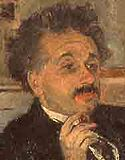Einstein's portrait by Harm Kamerlingh Onnes at the University of Leiden in 1920

In 1905, Albert Einstein took an extra step. He suggested that quantisation wasn't just a mathematical trick: the energy in a beam of light occurs in individual packets, which are now called photons. The energy of a single photon is given by its frequency multiplied by Planck's constant:$E = hf.\,$

For centuries, scientists had debated between two possible theories of light: was it a wave or did it instead comprise a stream of tiny particles? By the 19th century, the debate was generally considered to have been settled in favour of the wave theory, as it was able to explain observed effects such as refraction, diffraction and polarization. James Clerk Maxwell had shown that electricity, magnetism and light are all manifestations of the same phenomenon: the electromagnetic field. Maxwell's equations, which are the complete set of laws of classical electromagnetism, describe light as waves: a combination of oscillating electric and magnetic fields. Because of the preponderance of evidence in favour of the wave theory, Einstein's ideas were met initially by great scepticism. Eventually, however, the photon model became favoured; one of the most significant pieces of evidence in its favour was its ability to explain several puzzling properties of the photoelectric effect, described in the following section. Nonetheless, the wave analogy remained indispensable for helping to understand other characteristics of light, such as diffraction.

### The photoelectric effect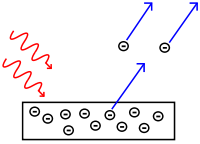Light (red arrows, left) is shone upon a metal. If the light is of sufficient frequency (i.e. sufficient energy), electrons are ejected (blue arrows, right).

In 1887 Heinrich Hertz observed that light can eject electrons from metal. In 1902 Philipp Lenard discovered that the maximum possible energy of an ejected electron is related to the frequency of the light, not to its intensity; if the frequency is too low, no electrons are ejected regardless of the intensity. The lowest frequency of light which causes electrons to be emitted, called the threshold frequency, is different for every metal. This is at odds with classical electromagnetism, which predicts that the electron's energy should be proportional to the intensity of the radiation.

Einstein explained the effect by postulating that a beam of light is a stream of particles (photons), and that if the beam is of frequency f each photon has an energy equal to hf (i.e. an integer multiple of Planck's constant). An electron is likely to be struck only by a single photon, which imparts at most an energy hf to the electron (in point of fact, it logically cannot be struck by more than one photon, since the first it absorbs will cause it to eject). Therefore, the intensity of the beam has no effect;[note 3] only its frequency determines the maximum energy that can be imparted to the electron.

To explain the threshold effect, Einstein argued that it takes a certain amount of energy, called the work function, denoted by φ, to remove an electron from the metal. This amount of energy is different for each metal. If the energy of the photon is less than the work function then it does not carry sufficient energy to remove the electron from the metal. The threshold frequency, f0, is the frequency of a photon whose energy is equal to the work function:$\varphi = h f_0.\,$

If f is greater than f0, the energy hf is enough to remove an electron. The ejected electron has a kinetic energy EK which is, at most, equal to the photon's energy minus the energy needed to dislodge the electron from the metal:

EK = hf − φ = h(ff0).

Einstein's description of light as being composed of particles extended Planck's notion of quantised energy: a single photon of a given frequency f delivers an invariant amount of energy hf. In other words, individual photons can deliver more or less energy, but only depending on their frequencies. However, although the photon is a particle it was still being described as having the wave-like property of frequency. Once again, the particle account of light was being "compromised".[note 4]

The relationship between the frequency of electromagnetic radiation and the energy of each individual photon is why ultraviolet light can cause sunburn, but visible or infrared light cannot. A photon of ultraviolet light will deliver a high amount of energy—enough to contribute to cellular damage such as a sunburn. A photon of infrared light will deliver a lower amount of energy—only enough to warm one's skin. So an infrared lamp can warm a large surface, perhaps large enough to keep people comfortable in a cold room, but it cannot give anyone a sunburn.

If each individual photon had identical energy, it would not be correct to talk of a "high energy" photon. Light of high frequency would carry more energy only because of a wave effect, i.e. because there were more photons arriving per second. If you doubled the frequency, you would double the number of energy units arriving each second: an argument based on intensity (i.e. on the number of photons per second). Einstein rejected that wave-dependent classical approach, in favour of a particle-based analysis where the energy of the particle must be absolute (since it is measured from a single impact only), and varies with frequency in discrete steps (i.e. is quantised). Hence he arrived at the concept of quantised energy levels.

In nature, single photons are not encountered. The sun emits photons continuously at all electromagnetic frequencies, so they appear to propagate as a continuous wave, not as discrete units. The emission sources available to Hertz and Lennard in the 19th century also had that characteristic. And the traditional mechanisms for generating photons are classical devices, in which the energy output is regulated by varying the frequency. But Einstein proposed that although a particular frequency is tied to a specific energy level, the frequency is dependent on the energy level, not vice versa (contrary to the tenets of classical physics). This formed his solution to the photoelectric effect, even though it was counter-intuitive.

And although the energy imparted by the photon is invariant at any given frequency, the initial energy-state of the electron prior to absorption is not. Therefore anomalous results may occur for individual electrons, but statistically a process of averaging will smooth out the results if a large enough number of electrons are emitted. This point is helpful in comprehending the distinction between the study of individual particles in quantum dynamics and the study of massed particles in classical physics.

## The quantisation of matter: the Bohr model of the atom

By the dawn of the 20th century, it was known that atoms comprise a diffuse cloud of negatively-charged electrons surrounding a small, dense, positively-charged nucleus. This suggested a model in which the electrons circle around the nucleus like planets orbiting a sun.[note 5] However, it was also known that the atom in this model would be unstable: according to classical theory orbiting electrons are undergoing centripetal acceleration, and should therefore give off electromagnetic radiation, the loss of energy also causing them to spiral toward the nucleus, colliding with it in a fraction of a second.

A second, related, puzzle was the emission spectrum of atoms. When a gas is heated, it gives off light only at discrete frequencies. For example, the visible light given off by hydrogen consists of four different colours, as shown in the picture below. By contrast, white light consists of a continuous emission across the whole range of visible frequencies.Emission spectrum of hydrogen. When excited, hydrogen gas gives off light in four distinct colours (spectral lines) in the visible spectrum, as well as a number of lines in the infra-red and ultra-violet.

In 1885 the Swiss mathematician Johann Balmer discovered that each wavelength λ (lambda) in the visible spectrum of hydrogen is related to some integer n by the equation$\lambda = B\left(\frac{n^2}{n^2-4}\right) \qquad\qquad n = 3,4,5,6$

where B is a constant which Balmer determined to be equal to 364.56 nm. Thus Balmer's constant was the basis of a system of discrete, i.e. quantised, integers.

In 1888 Johannes Rydberg generalized and greatly increased the explanatory utility of Balmer's formula. He predicted that hydrogen will emit light of wavelength λ (lambda) where λ is related to two integers n and m according to what is now known as the Rydberg formula:$\frac{1}{\lambda} = R \left(\frac{1}{m^2} - \frac{1}{n^2}\right),$

where R is the Rydberg constant, equal to 0.0110 nm−1, and n must be greater than m.

Rydberg's formula accounts for the four visible wavelengths of hydrogen by setting m = 2 and n = 3,4,5,6. It also predicts additional wavelengths in the emission spectrum: for m = 1 and for n > 1, the emission spectrum should contain certain ultraviolet wavelengths, and for m = 3 and n > 3, it should also contain certain infrared wavelengths. Experimental observation of these wavelengths came two decades later: in 1908 Louis Paschen found some of the predicted infrared wavelengths, and in 1914 Theodore Lyman found some of the predicted ultraviolet wavelengths.

### Bohr's model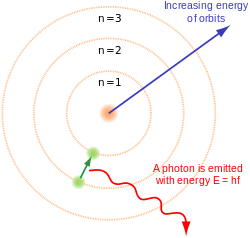The Bohr model of the atom, showing an electron quantum jumping to ground state n = 1.

In 1913 Niels Bohr proposed a new model of the atom that included quantized electron orbits. In Bohr's model, electrons could inhabit only certain orbits around the atomic nucleus. When an atom emitted (or absorbed) energy, the electron did not move in a continuous trajectory from one orbit around the nucleus to another, as might be expected classically. Instead, the electron would jump instantaneously from one orbit to another, giving off the emitted light in the form of a photon. The possible energies of photons given off by each element were determined by the differences in energy between the orbits, and so the emission spectrum for each element would contain a number of lines.

Bohr theorised that the angular momentum, L, of an electron is quantised:$L = n\frac{h}{2\pi},$

where n is an integer and h is the Planck constant. Starting from this assumption, Coulomb's law and the equations of circular motion show that an electron with n units of angular momentum will orbit a proton at a distance r given by$r = \frac{n^2 h^2}{4 \pi^2 k_e m e^2}$,

where ke is the Coulomb constant, m is the mass of an electron, and e is the charge on an electron. For simplicity this is written as$r = n^2 a_0,\!$

where a0, called the Bohr radius, is equal to 0.0529 nm. The Bohr radius is the radius of the smallest allowed orbit.

The energy of the electron[note 6] can also be calculated, and is given by$E = -\frac{k_{\mathrm{e}}e^2}{2a_0} \frac{1}{n^2}$.

Thus Bohr's assumption that angular momentum is quantised means that an electron can only inhabit certain orbits around the nucleus, and that it can have only certain energies. A consequence of these constraints is that the electron will not crash into the nucleus: it cannot continuously emit energy, and it cannot come closer to the nucleus than a0 (the Bohr radius).

An electron loses energy by jumping instantaneously from its original orbit to a lower orbit; the extra energy is emitted in the form of a photon. Conversely, an electron that absorbs a photon gains energy, hence it jumps to an orbit that is farther from the nucleus.

Each photon from glowing atomic hydrogen is due to an electron moving from a higher orbit, with radius rn, to a lower orbit, rm. The energy Eγ of this photon is the difference in the energies En and Em of the electron:$E_{\gamma} = E_n - E_m = \frac{k_{\mathrm{e}}e^2}{2a_0}\left(\frac{1}{m^2}-\frac{1}{n^2}\right)$

Since Planck's equation shows that the photon's energy is related to its wavelength by Eγ = hc/λ, the wavelengths of light which can be emitted are given by$\frac{1}{\lambda} = \frac{k_{\mathrm{e}}e^2}{2 a_0 h c}\left(\frac{1}{m^2}-\frac{1}{n^2}\right).$

This equation has the same form as the Rydberg formula, and predicts that the constant R should be given by$R = \frac{k_{\mathrm{e}}e^2}{2 a_0 h c} .$

Therefore the Bohr model of the atom can predict the emission spectrum of hydrogen in terms of fundamental constants.[note 7] However, it was not able to make accurate predictions for multi-electron atoms, or to explain why some spectral lines are brighter than others.

## Wave-particle duality

In 1924, Louis de Broglie proposed the idea that just as light has both wave-like and particle-like properties, matter also has wave-like properties. The wavelength, λ, associated with a particle is related to its momentum, p:$p = \frac{h}{\lambda}.$

The relationship, called the de Broglie hypothesis, holds for all types of matter. Thus all matter exhibits properties of both particles and waves.

Three years later, the wave-like nature of electrons was demonstrated by showing that a beam of electrons could exhibit diffraction, just like a beam of light. At the University of Aberdeen, George Thomson passed a beam of electrons through a thin metal film and observed the predicted interference patterns. At Bell Labs, Davisson and Germer guided their beam through a crystalline grid. Similar wave-like phenomena were later shown for atoms and even small molecules. De Broglie was awarded the Nobel Prize for Physics in 1929 for his hypothesis; Thomson and Davisson shared the Nobel Prize for Physics in 1937 for their experimental work.

This is the concept of wave-particle duality: neither the classical concepts of "particle" or "wave" can fully describe the behavior of quantum-scale objects, either photons or matter. Wave-particle duality is an example of the principle of complementarity in quantum physics. An elegant example of wave-particle duality, the double slit experiment, is discussed in the section below.

De Broglie's treatment of quantum events served as a jumping off point for Schrödinger when he set about to construct a wave equation to describe quantum theoretical events.

### The double-slit experimentLight from one slit interferes with light from the other, producing an interference pattern (the 3 fringes shown at the right).

In the double-slit experiment as originally performed by Thomas Young and Augustin Fresnel in 1827, a beam of light is directed through two narrow, closely spaced slits, producing an interference pattern of light and dark bands on a screen. If one of the slits is covered up, one might naively expect that the intensity of the fringes due to interference would be halved everywhere. In fact, a much simpler pattern is seen, a simple diffraction pattern. Closing one slit results in a much simpler pattern diametrically opposite the open slit. Exactly the same behaviour can be demonstrated in water waves, and so the double-slit experiment was seen as a demonstration of the wave nature of light.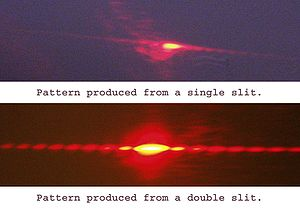The diffraction pattern produced when light is shone through one slit (top) and the interference pattern produced by two slits (bottom). The interference pattern from two slits is much more complex, demonstrating the wave-like propagation of light.

The double-slit experiment has also been performed using electrons, atoms, and even molecules, and the same type of interference pattern is seen. Thus all matter possesses both particle and wave characteristics.

Even if the source intensity is turned down so that only one particle (e.g. photon or electron) is passing through the apparatus at a time, the same interference pattern develops over time. The quantum particle acts as a wave when passing through the double slits, but as a particle when it is detected. This is a typical feature of quantum complementarity: a quantum particle will act as a wave when we do an experiment to measure its wave-like properties, and like a particle when we do an experiment to measure its particle-like properties. Where on the detector screen any individual particle shows up will be the result of an entirely random process.

### Application to the Bohr model

De Broglie expanded the Bohr model of the atom by showing that an electron in orbit around a nucleus could be thought of as having wave-like properties. In particular, an electron will be observed only in situations that permit a standing wave around a nucleus. An example of a standing wave is a violin string, which is fixed at both ends and can be made to vibrate. The waves created by a stringed instrument appear to oscillate in place, moving from crest to trough in an up-and-down motion. The wavelength of a standing wave is related to the length of the vibrating object and the boundary conditions. For example, because the violin string is fixed at both ends, it can carry standing waves of wavelengths 2l/n, where l is the length and n is a positive integer. De Broglie suggested that the allowed electron orbits were those for which the circumference of the orbit would be an integer number of wavelengths.

## Development of modern quantum mechanics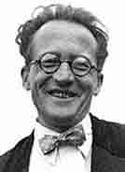Erwin Schrödinger, about 1933, age 46

In 1925, building on de Broglie's hypothesis, Erwin Schrödinger developed the equation that describes the behaviour of a quantum mechanical wave. The equation, called the Schrödinger equation after its creator, is central to quantum mechanics, and defines the permitted stationary states of a quantum system, and describes how the quantum state of a physical system changes in time.

Schrödinger was able to calculate the energy levels of hydrogen by treating a hydrogen atom's electron as a classical wave, moving in a well of electrical potential created by the proton. This calculation accurately reproduced the energy levels of the Bohr model.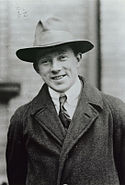Werner Heisenberg at the age of 26. Heisenberg won the Nobel Prize in Physics in 1932 for the work that he did at around this time.

At around the same time, Werner Heisenberg was trying to find an explanation for the intensities of the different lines in the hydrogen emission spectrum. By means of a series of mathematical analogies, Heisenberg wrote out the quantum mechanical analogue for the classical computation of intensities. Shortly afterwards, Heisenberg's colleague Max Born realised that Heisenberg's method of calculating the probabilities for transitions between the different energy levels could best be expressed by using the mathematical concept of matrices.[note 8]

In May 1926, Schrödinger proved that Heisenberg's matrix mechanics and his own wave mechanics made the same predictions about the properties and behaviour of the electron; mathematically, the two theories were identical. Yet the two men disagreed on the interpretation of their mutual theory. Heisenberg saw no problem in the existence of discontinuous quantum jumps, but Schrödinger hoped that a theory based on continuous wave-like properties could avoid what he called (in the words of Wilhelm Wien) "this nonsense about quantum jumps."

## Copenhagen interpretation

Bohr, Heisenberg and others tried to explain what these experimental results and mathematical models really mean. Their description, known as the Copenhagen interpretation of quantum mechanics, aimed to describe the nature of reality that was being probed by the measurements and described by the mathematical formulations of quantum mechanics.

The main principles of the Copenhagen interpretation are:

1. A system is completely described by a wave function, ψ. (Heisenberg)
2. How ψ changes over time is given by the Schrödinger equation.
3. The description of nature is essentially probabilistic. The probability of an event — for example, where on the screen a particle will show up in the two slit experiment — is related to the square of the amplitude of its wave function. (Born rule, due to Max Born, which gives a physical meaning to the wavefunction in the Copenhagen interpretation: the probability amplitude)
4. It is not possible to know the values of all of the properties of the system at the same time; those properties that are not known with precision must be described by probabilities. (Heisenberg's uncertainty principle)
5. Matter, like energy, exhibits a wave-particle duality. An experiment can demonstrate the particle-like properties of matter, or its wave-like properties; but not both at the same time. (Complementarity principle due to Bohr)
6. Measuring devices are essentially classical devices, and measure classical properties such as position and momentum.
7. The quantum mechanical description of large systems should closely approximate the classical description. (Correspondence principle of Bohr and Heisenberg)

Various consequences of these principles are discussed in more detail in the following subsections.

### Uncertainty principle

Suppose that we want to measure the position and speed of an object — for example a car going through a radar speed trap. Naively, we assume that the car has a definite position and speed at a particular moment in time, and how accurately we can measure these values depends on the quality of our measuring equipment — if we improve the precision of our measuring equipment, we will get a result that is closer to the true value. In particular, we would assume that how precisely we measure the speed of the car does not affect its position, and vice versa.

In 1927, Heisenberg proved that these assumptions are not correct. Quantum mechanics shows that certain pairs of physical properties, like position and speed, cannot both be known to arbitrary precision: the more precisely one property is known, the less precisely the other can be known. This statement is known as the uncertainty principle. The uncertainty principle isn't a statement about the accuracy of our measuring equipment, but about the nature of the system itself — our naive assumption that the car had a definite position and speed was incorrect. On a scale of cars and people, these uncertainties are too small to notice, but when dealing with atoms and electrons they become critical.

The uncertainty principle shows mathematically that the product of the uncertainty in the position and momentum of a particle (momentum is velocity multiplied by mass) could never be less than a certain value, and that this value is related to Planck's constant.

### Wave function collapse

Wave function collapse is a forced term for whatever happened when it becomes appropriate to replace the description of an uncertain state of a system by a description of the system in a definite state. Explanations for the nature of the process of becoming certain are controversial. At any time before a photon "shows up" on a detection screen it can only be described by a set of probabilities for where it might show up. When it does show up, for instance in the CCD of an electronic camera, the time and the space where it interacted with the device are known within very tight limits. However, the photon has disappeared, and the wave function has disappeared with it. In its place some physical change in the detection screen has appeared, e.g., an exposed spot in a sheet of photographic film.

### Eigenstates and eigenvalues

For a more detailed introduction to this subject, see: Introduction to eigenstates

Because of the uncertainty principle, statements about both the position and momentum of particles can only assign a probability that the position or momentum will have some numerical value. Therefore it is necessary to formulate clearly the difference between the state of something that is indeterminate, such as an electron in a probability cloud, and the state of something having a definite value. When an object can definitely be "pinned-down" in some respect, it is said to possess an eigenstate.

### The Pauli exclusion principle

Wolfgang Pauli proposed the following concise statement of his 1925 principle: "There cannot exist an atom in such a quantum state that two electrons within [it] have the same set of quantum numbers."

He developed the exclusion principle from what he called a "two-valued quantum degree of freedom" to account for the observation of a doublet, meaning a pair of lines differing by a small amount (e.g. on the order of 0.15 Å), in the spectrum of atomic hydrogen. The existence of these closely spaced lines in the bright-line spectrum meant that there was more energy in the electron orbital from magnetic moments than had previously been described.

In early 1925, Uhlenbeck and Goudsmit proposed that electrons rotate about an axis in the same way that the earth rotates on its axis. They proposed to call this property spin. Spin would account for the missing magnetic moment, and allow two electrons in the same orbital to occupy distinct quantum states if they, "spun" in opposite directions, thus satisfying the Exclusion Principle. A new quantum number was then needed, one to represent the momentum embodied in the rotation of each electron.

### Application to the hydrogen atom

Schrödinger was able to calculate the energy levels of hydrogen by treating a hydrogen atom's electron as a wave, represented by the "wave function" Ψ, in a electric potential well, V, created by the proton. In Bohr's theory, electrons orbited the nucleus much as planets orbit the sun. Schrödinger's model gives the probability of the electron being located at any particular location – following the uncertainty principle, the electron cannot be viewed as having an exact location at any given time. The solutions to Schrödinger's equation are called atomic orbitals, "clouds" of possible locations in which an electron might be found, a distribution of probabilities rather than a precise location. Orbitals have a range of different shapes in three dimensions. The energies of the different orbitals can be calculated, and they accurately reproduce the energy levels of the Bohr model.

Within Schrödinger's picture, each electron has four properties:

1. An "orbital" designation, indicating whether the particle wave is one that is closer to the nucleus with less energy or one that is farther from the nucleus with more energy;
2. The "shape" of the orbital, spherical or otherwise;
3. The "inclination" of the orbital, determining the magnetic moment of the orbital around the z-axis.
4. The "spin" of the electron.

The collective name for these properties is the quantum state of the electron. The quantum state can be described by giving a number to each of these properties; these are known as the electron's quantum numbers. The quantum state of the electron is described by its wavefunction. The Pauli exclusion principle demands that no two electrons within an atom may have the same values of all four numbers.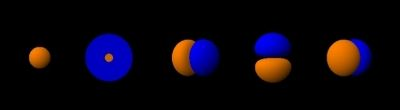The shapes of the first five atomic orbitals: 1s, 2s, 2px,2py, and 2pz. The colours show the phase of the wavefunction.

The first property describing the orbital is the principal quantum number, n, which is the same as in Bohr's model. n denotes the energy level of each orbital. The possible values for n are integers:$n = 1, 2, 3\ldots$

The next quantum number, the azimuthal quantum number, denoted l, describes the shape of the orbital. The shape is a consequence of the angular momentum of the orbital. The angular momentum represents the resistance of a spinning object to speeding up or slowing down under the influence of external force. The azimuthal quantum number represents the orbital angular momentum of an electron around its nucleus. The possible values for l are integers from 0 to n − 1:$l = 0, 1, \ldots, n-1$. The shape of each orbital has its own letter as well. The first shape is denoted by the letter s (a mnemonic being "sphere"). The next shape is denoted by the letter p and has the form of a dumbbell. The other orbitals have more complicated shapes (see atomic orbital), and are denoted by the letters d, f, and g.

The third quantum number, the magnetic quantum number, describes the magnetic moment of the electron, and is denoted by ml (or simply m). The possible values for ml are integers from −l to l:$m_l = -l, -(l-1), \ldots, 0, 1, \ldots, l$. The magnetic quantum number measures the component of the angular momentum in a particular direction. The choice of direction is arbitrary, conventionally the z-direction is chosen.

The fourth quantum number, the spin quantum number (pertaining to the "orientation" of the electron's spin) is denoted ms, with values +12 or −12.

The chemist Linus Pauling wrote, by way of example:

In the case of a helium atom with two electrons in the 1 s orbital, the Pauli Exclusion Principle requires that the two electrons differ in the value of one quantum number. Their values of n, l, and ml are the same; moreover, they have the same spin, s = 12. Accordingly they must differ in the value of ms, which can have the value of +12 for one electron and −12 for the other."

It is the underlying structure and symmetry of atomic orbitals, and the way that electrons fill them, that determines the organisation of the periodic table and the structure and strength of chemical bonds between atoms.

## Dirac wave equation

In 1928, Paul Dirac extended the Pauli equation, which described spinning electrons, to account for special relativity. The result was a theory that dealt properly with events, such as the speed at which an electron orbits the nucleus, occurring at a substantial fraction of the speed of light. By using the simplest electromagnetic interaction, Dirac was able to predict the value of the magnetic moment associated with the electron's spin, and found the experimentally observed value, which was too large to be that of a spinning charged sphere governed by classical physics. He was able to solve for the spectral lines of the hydrogen atom, and to reproduce from physical first principles Sommerfeld's successful formula for the fine structure of the hydrogen spectrum.

Dirac's equations sometimes yielded a negative value for energy, for which he proposed a novel solution: he posited the existence of an antielectron and of a dynamical vacuum. This led to many-particle quantum field theory.

## Quantum entanglementSuperposition of two quantum characteristics, and two resolution possibilities.

The Pauli exclusion principle says that two electrons in one system cannot be in the same state. Nature leaves open the possibility, however, that two electrons can have both states "superimposed" over them. Recall that the wave functions that emerge simultaneously from the double slits arrive at the detection screen in a state of superposition. Nothing is certain until the superimposed waveforms "collapse," At that instant an electron shows up somewhere in accordance with the probabilities that are the squares of the amplitudes of the two superimposed waveforms. The situation there is already very abstract. A concrete way of thinking about entangled photons, photons in which two contrary states are superimposed on each of them in the same event, is as follows:

Imagine that the superposition of a state that can be mentally labeled as blue and another state that can be mentally labeled as red will then appear (in imagination, of course) as a purple state. Two photons are produced as the result of the same atomic event. Perhaps they are produced by the excitation of a crystal that characteristically absorbs a photon of a certain frequency and emits two photons of half the original frequency. So the two photons come out "purple." If the experimenter now performs some experiment that will determine whether one of the photons is either blue or red, then that experiment changes the photon involved from one having a superposition of "blue" and "red" characteristics to a photon that has only one of those characteristics. The problem that Einstein had with such an imagined situation was that if one of these photons had been kept bouncing between mirrors in a laboratory on earth, and the other one had traveled halfway to the nearest star, when its twin was made to reveal itself as either blue or red, that meant that the distant photon now had to lose its "purple" status too. So whenever it might be investigated, it would necessarily show up, instantaneously, in the opposite state to whatever its twin had revealed.

In trying to show that quantum mechanics was not a complete theory, Einstein started with the theory's prediction that two or more particles that have interacted in the past can appear strongly correlated when their various properties are later measured. He sought to explain this seeming interaction in a classical way, through their common past, and preferably not by some "spooky action at a distance." The argument is worked out in a famous paper, Einstein, Podolsky, and Rosen (1935; abbreviated EPR), setting out what is now called the EPR paradox. Assuming what is now usually called local realism, EPR attempted to show from quantum theory that a particle has both position and momentum simultaneously, while according to the Copenhagen interpretation, only one of those two properties actually exists and only at the moment that it is being measured. EPR concluded that quantum theory is incomplete in that it refuses to consider physical properties which objectively exist in nature. (Einstein, Podolsky, & Rosen 1935 is currently Einstein's most cited publication in physics journals.) In the same year, Erwin Schrödinger used the word "entanglement" and declared: "I would not call that one but rather the characteristic trait of quantum mechanics."  The question of whether entanglement is a real condition is still in dispute. The Bell inequalities are the most powerful challenge to Einstein's claims.

## Quantum electrodynamics

Quantum electrodynamics (QED) is the name of the quantum theory of the electromagnetic force. Understanding QED begins with understanding electromagnetism. Electromagnetism can be called "electrodynamics" because it is a dynamic interaction between electrical and magnetic forces. Electromagnetism begins with the electric charge.

Electric charges are the sources of, and create, electric fields. An electric field is a field which exerts a force on any particles that carry electric charges, at any point in space. This includes the electron, proton, and even quarks, among others. As a force is exerted, electric charges move, a current flows and a magnetic field is produced. The magnetic field, in turn causes electric current (moving electrons). The interacting electric and magnetic field is called an electromagnetic field.

The physical description of interacting charged particles, electrical currents, electrical fields, and magnetic fields is called electromagnetism.

In 1928 Paul Dirac produced a relativistic quantum theory of electromagnetism. This was the progenitor to modern quantum electrodynamics, in that it had essential ingredients of the modern theory. However, the problem of unsolvable infinities developed in this relativistic quantum theory. Years later, renormalization solved this problem. Initially viewed as a suspect, provisional procedure by some of its originators, renormalization eventually was embraced as an important and self-consistent tool in QED and other fields of physics. Also, in the late 1940s Feynman's diagrams showed all possible interactions of a given event. The diagrams showed that the electromagnetic force is the interactions of photons between interacting particles.

An example of a prediction of quantum electrodynamics which has been verified experimentally is the Lamb shift. This refers to an effect whereby the quantum nature of the electromagnetic field causes the energy levels in an atom or ion to deviate slightly from what they would otherwise be. As a result, spectral lines may shift or split.

In the 1960s physicists realized that QED broke down at extremely high energies. From this inconsistency the Standard Model of particle physics was discovered, which remedied the higher energy breakdown in theory. The Standard Model unifies the electromagnetic and weak interactions into one theory. This is called the electroweak theory.

## Interpretations

The physical measurements, equations, and predictions pertinent to quantum mechanics are all consistent and hold a very high level of confirmation. However, the question of what these abstract models say about the underlying nature of the real world has received competing answers.

 Coherence Experiments: Glossary of elementary quantum mechanics History of quantum mechanics Heisenberg's entryway to matrix mechanics Interpretations: Measurement in quantum mechanics Measurement problem Orbital:

Persons important for discovering and elaborating quantum theory:

 Hugh Everett Markus Fierz

Wikimedia Foundation. 2010.

### Look at other dictionaries:

• Quantum mechanics — For a generally accessible and less technical introduction to the topic, see Introduction to quantum mechanics. Quantum mechanics …   Wikipedia

• quantum mechanics — quantum mechanical, adj. Physics. a theory of the mechanics of atoms, molecules, and other physical systems that are subject to the uncertainty principle. Abbr.: QM Cf. nonrelativistic quantum mechanics, relativistic quantum mechanics. [1920 25]… …   Universalium

• Measurement in quantum mechanics — Quantum mechanics Uncertainty principle …   Wikipedia

• P-adic quantum mechanics — One may compute the energy levels for a potential well like this one.[note 1] P adic quantum mechanics is a relatively recent approach to understanding the nature of fundamental physics. It is the application of p adic analysis to quantum… …   Wikipedia

• History of quantum mechanics — The history of quantum mechanics as this interlaces with history of quantum chemistry began essentially with the 1838 discovery of cathode rays by Michael Faraday, during the 1859 1860 winter statement of the black body radiation problem by… …   Wikipedia

• Variational method (quantum mechanics) — The variational method is, in quantum mechanics, one way of finding approximations to the lowest energy eigenstate or ground state, and some excited states. The basis for this method is the variational principle. Introduction Suppose we are given …   Wikipedia